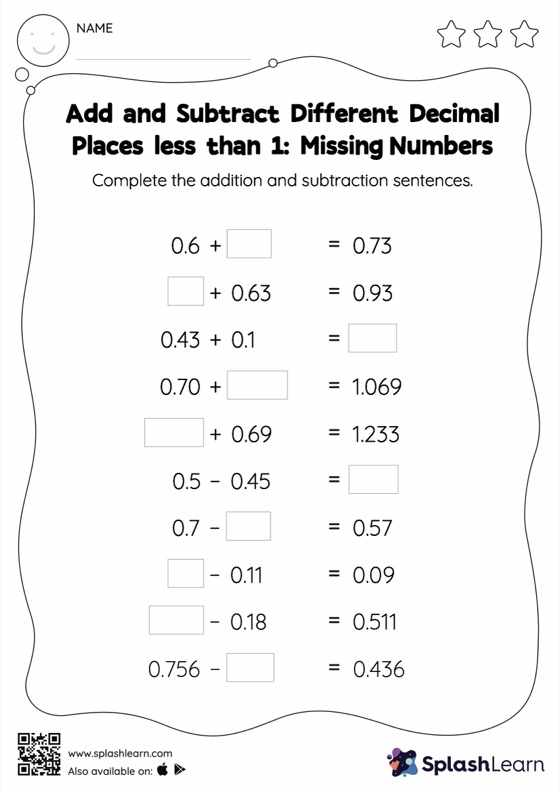# Add and Subtract Different Decimal Places less than 1: Missing Numbers Worksheet

Home > Add and Subtract Different Decimal Places less than 1: Missing NumbersHelp your little one develop a knack for math with this add and subtract different decimal places less than 1 worksheet. In this worksheet, students add and subtract decimals by aligning the decimal points and using zero as a placeholder. They then use the relationship between addition and subtraction to find the missing number in the add and subtract different decimal places less than 1 worksheet.